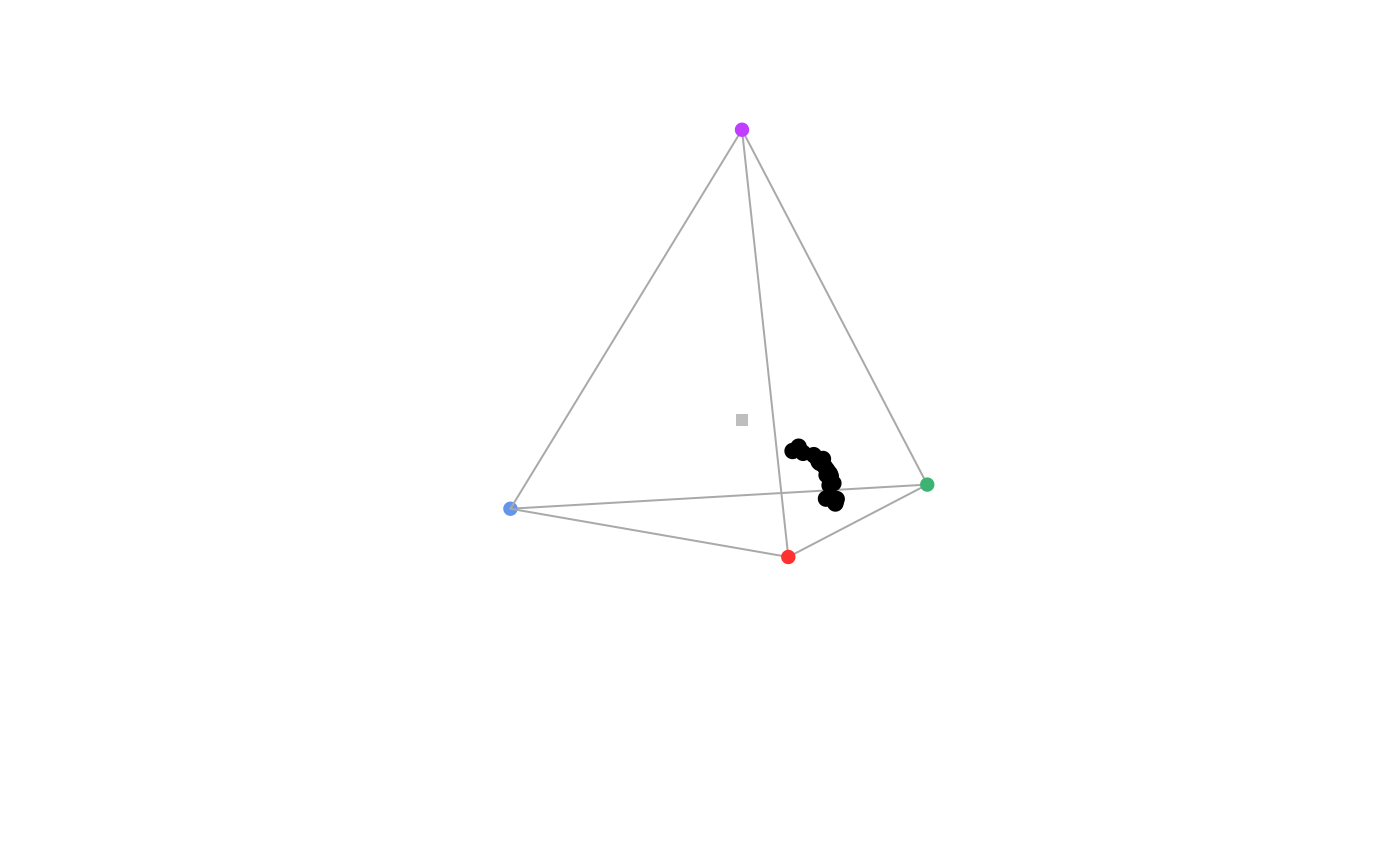Plots reference x, y and z arrows showing the direction of the axes in a static tetrahedral colourspace plot.

axistetra(
x = 0,
y = 1.3,
size = 0.1,
col = par("fg"),
lty = par("lty"),
lwd = par("lwd"),
label = TRUE,
adj.label = list(x = c(0.003, 0), y = c(0.003, 0.003), z = c(0, 0.003)),
label.cex = 1,
label.col = NULL
)

## Arguments

x, y

position of the legend relative to plot limits (usually a value between 0 and 1, but because of the perspective distortion, values greater than 1 are possible)

size

length of the arrows. Can be either a single value (applied for x, y and z) or a vector of 3 separate values for each axis.

col, lty, lwd

graphical parameters for the arrows.

label

logical, include x, y and z labels (defaults to TRUE).

position adjustment for the labels. a list of 3 named objects for x, y and z arrows, each with 2 values for x and y adjustment.

label.cex, label.col

graphical parameters for the labels.

## Value

axistetra adds reference arrows showing the direction of the 3-dimensional axes in a static tetrahedral colourspace plot.

## Author

Rafael Maia rm72@zips.uakron.edu

## Examples

data(sicalis)
vis.sicalis <- vismodel(sicalis, visual = "avg.uv")
tcs.sicalis <- colspace(vis.sicalis, space = "tcs")
plot(tcs.sicalis)
axistetra()